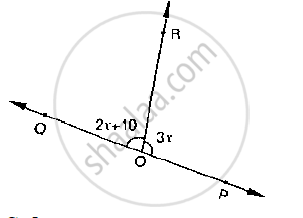# Given ∠Por = 3x and ∠Qor = 2x + 10, Find the Value of X for Which Poq Will Be a Line. (Below Fig). - Mathematics

Given ∠POR = 3x and ∠QOR = 2x + 10, find the value of x for which POQ will be a line.
(Below fig).#### Solution

Since ∠QOR, ∠POP are linear pairs

∠QOR + ∠POR = 180°

2x +10 + 3x = 180°                    [ ∴ ∠QOR = 2x +10, ∠POR = 3x]

⇒ 5x +10 = 180°

⇒ 5x = 180° -10

5x = 170°

⇒ x = 34°

Concept: Pairs of Angles
Is there an error in this question or solution?
Chapter 10: Lines and Angles - Exercise 10.2 [Page 15]

#### APPEARS IN

RD Sharma Mathematics for Class 9
Chapter 10 Lines and Angles
Exercise 10.2 | Q 12 | Page 15

Share